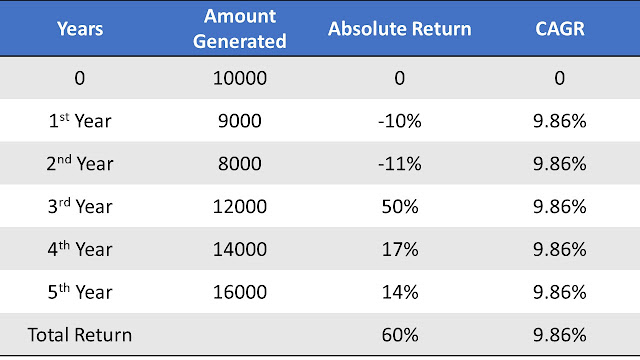### BN1# CAGR % - Compound Annual Growth Rate

CAGR% - Compound Annual Growth Rate

Get Connect to : themarketwarriorABSOLUTE RETURN Vs CAGR

The main moto of our investment is to get maximum return. And everyone excited to get more and more return on their investments. But people are totally confused or unware, how to measure their investment returns. Whether to calculate return in absolute return or CAGR.

So, lets go and learn CAGR,

What is CAGR (Compound Annual Growth Rate)?

The compound annual growth rate (CAGR) is a average annual growth measurement of your investments over a fixed time period. It give you clear picture of the average rate of return on your assets over the course of a year.

And it is measure in terms of % i.e CAGR%.

How CAGR is calculated (mathematical formula)?

CAGR = {(Final Amount / Initial Amount)^(1/n)}-1

n = no of years,

For example:

* Initial amount invested - \$10000

* Final amount generate in 5 years - \$16000

* n = 5 years

then,

CAGR = {(16000/10000)^(1/5)}-1

CAGR = 9.86 %.

So, how the Absolute Return and CAGR are different?

Let's again use same above example

* Initial amount invested - \$10000

* Final amount generate in 5 years - \$16000

* n = 5 years

Absolute return

= (Final amount - Initial amount)/ Initial amount * 100

= (16000-10000)/10000 * 100

= (6000/10000) * 100

= 60%.

Here in Absolute return there is no relation shown with the investment years, only initial amount (\$10000) and final amount (\$16000) taken into consideration for calculation of return.

Whereas in CAGR average out return is calculated, as in our above example of CARG return in 5 years is 9.86% means every year return is calculated with 9.86%,

1st year return is 9.86%

2nd year return is 9.86%

3rd year return is 9.86%

4th year return is 9.86%

5th year return is 9.86%

Return is similar in all years.5 YEARS ABSOLUTE RETURN & CAGRABSOLUTE RETURN VS CAGR

Important point to remember,

Care to be taken to calculate the CAGR with proper duration:

For example,

Initial investment (0 Year ) - \$10000

1st year - \$9000

2nd year - \$8000

3rd year - \$9500

4th year - \$11000

5th year - \$13000

So,

initial 3 years CAGR =  (-1.7)%

3 years CAGR

= {(9500/10000)^(1/3)}-1

= (-1.7)%

last 3 years CARG = 17.57%

3 years CAGR

= {(13000/8000)^(1/3)}-1

= 17.57%

5 years CAGR = 5.39%

= {(13000/10000)^(1/5)}-1

= 5.39%

Conclusion

It's time to wrap up. Basically in financial matter investment return  calculation should be  systematic addressed. Absolute return doesn't give clear picture of investment return with respect to duration (no of years of investment), which is very important fact. Whereas the calculation of investment return with CAGR consider the period of investment for calculating investment return.

Don't Forget to LIKE, FOLLOW, SUBSCRIBE & SHARE with your Friend's & Family.

For Shopping & Deals You Can Visit :   https://bestchoice4every1.blogspot.com

1.